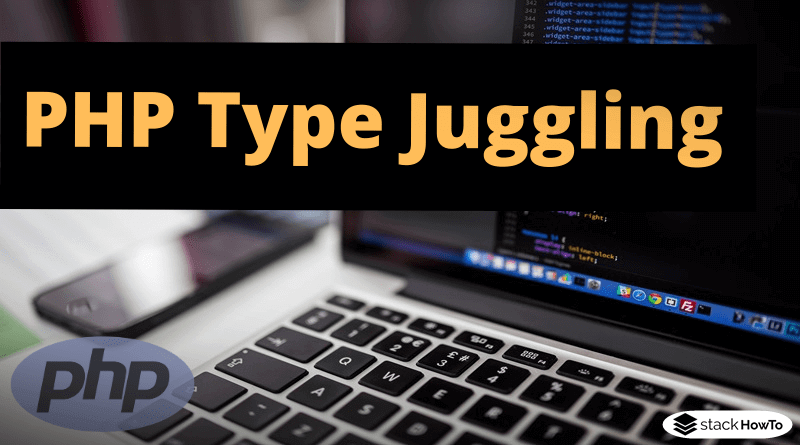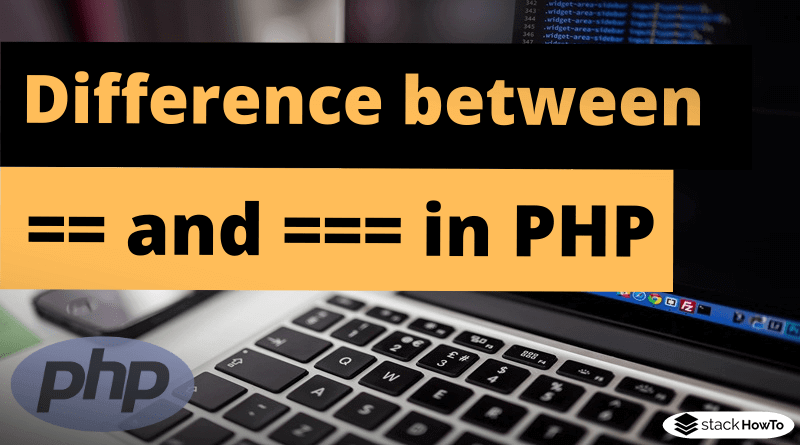php

# Type casting in PHP

Type Cast is the conversion of a variable of data type A into another data type B. For example, converting an integer variable into a float variable would be a typecast. PHP supports type casts primarily for primitive data types (integer, float, Boolean, …) and is very tolerant in this area so that strings can be converted into integers. Unlike Java, however, type casts from one class A to another class B are not supported – even if A inherits from B.

In order to perform a type cast, the new data type must be written in brackets in front of the variable: `(new_datype)\$variable`. The following casts are possible:

(int) and (integer): conversion to integer.

(float), (double) and (real): conversion to float.

(string): conversion to string.

(bool) and (boolean): conversion to Boolean.

(array): conversion into an array. When applied to a variable, an array is usually generated, which in turn contains the variable as the only value.

(object): conversion into an object of the class stdClass. When applied to Float, Integer, String or Boolean, the resulting object has a field called “scalar” which contains the variable. When applied to an array or to NULL, an stdClass object without fields is generated. When applied to an object, the object remains unchanged.

(unset): sets the variable to NULL, so it is identical to \$variable = null;

(binary): conversion of strings into binary strings.PHP Questions and Answers – Object-Oriented OOP – Part 1This collection of PHP Multiple Choice Questions and Answers (MCQs): Quizzes & Practice Tests with Answer focuses on “Object-Oriented OOP”.   1. The concept of…Read More

###### Example 1: Type cast from Integer to Float

In this example, a variable of the type integer is converted into a float variable.

```<?php
\$a = 5;
\$b = (float)\$a;
var_dump(\$a, \$b);
?>```

Output:

```int(5)
float(5)```

###### Example 2: Type cast from String to Integer or (int)

In this example, a variable of the type String is converted into an integer.

```<?php
\$a = '5';
\$b = (int)\$a;
var_dump(\$a, \$b);
?>```

Output:

```string(1) "5"
int(5)```

When converting from string to integer, all numbers from the beginning of the string to the first character that is not in the range 0 to 9 are taken.

```<?php
\$a = '55xyz'; // 55 is taken over from this string
\$b = 'xyz55'; // nothing will be taken over from this string
var_dump((int)\$a, (int)\$b);
?>```

Output:

```int(55)
int(0)```PHP Type JugglingType juggling refers to PHP’s ability to automatically change the data types of variables depending on the context in which they are used.   Example…Read More
###### Example 3: Type cast from Integer to Boolean or (bool)

Conversion of integer values to Boolean. The number 0 is interpreted as false, all other values as true.

```<?php
\$a = 0;
\$b = 1;
\$c = 5;
\$d = -5;
var_dump((bool)\$a, (bool)\$b, (bool)\$c, (bool)\$d);
?>```

Output:

```bool(false)
bool(true)
bool(true)
bool(true)```

###### Example 4: Type cast from Float to String

Representation of the conversion from float to string. If you don’t want to have the decimal places, `round(\$var, \$precision)` must be used, where \$precision corresponds to the number of decimal places.

```<?php
\$a = 1/6;
\$b = (string)\$a;
var_dump(\$a, \$b);
?>```

Output:

```float(0.16666666666667)
string(16) "0.16666666666667"```What is the difference between == and === in PHPIn this tutorial, we’re going to see two ways to compare variables for equality in PHP.   The general behavior of “==” In most programming…Read More
###### Example 5: Type cast from Float to Array

Conversion of a float value into an array. An array is created, which contains the float value as the only element.

```<?php
\$a = 5.99;
\$b = (array)\$a;
var_dump(\$a, \$b);
?>```

Output:

```float(5.99)
array(1) {
=>
float(5.99)
}```

###### Example 6: Type cast from String to NULL

Conversion from string to NULL, identical to \$a = null.

```<?php
\$a = 'test';
\$b = (unset)\$a;
var_dump(\$a, \$b);
?>```

Output:

```string(4) "test"
NULL```

###### Example 7: Type cast from String to Object

In this example a string is converted into an object of the class stdClass.

```<?php
\$a = 'test';
\$b = (object)\$a;
var_dump(\$a, \$b);
?>```

Output:

```string(4) "test"
object(stdClass)#1 (1) {
["scalar"]=>
string(4) "test"
}```How to check if a variable is an Integer in PHP?Which methods are available in PHP to check if a variable is an Integer? In this tutorial, we are going to see how to check…Read More
###### Example 8: Type cast between classes is not possible

A type cast between different classes is not possible, even if they belong to the same inheritance hierarchy.

```<?php
class A { }
class B { }

\$b = new B();
\$a = (A)\$b;
?>```

Executing the code produces a Type Cast error, as expected:

Output:

`Parse error:  syntax error, unexpected '\$b' (T_VARIABLE) in [...][...] on line 6`MCQPractice competitive and technical Multiple Choice Questions and Answers (MCQs) with simple and logical explanations to prepare for tests and interviews.Read More